# EA is opening trades when the conditions are not met - How to prevent that ?815

Hello MQL community,

I just started to learn the MQL language and I am currently trying to build my first EA.

So in my first EA I want to only do a CALL or PUT when the fractal+diamond occurred two candlesticks apart (See image for example)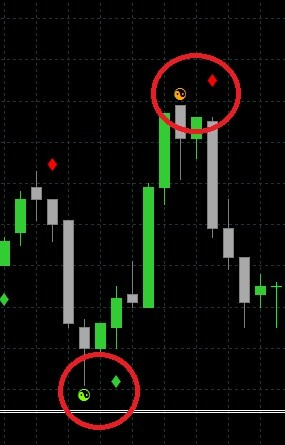If the Fractal (the yingyang symbol is green) The Value 2 in the Data Window in MT4 shows a value: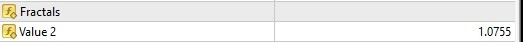If the Fractal (the yingyang symbol is orange) The Fractals Colmun in the Data Window in MT4 shows a value: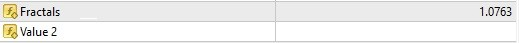If a diamond in green appears The M1LWMA 4x4 show a value: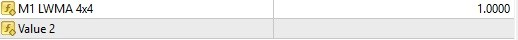If diamond in red appears the Value 2 shows a value.

Based on that I tried to come up with the correct logic in the code. Below you can see my attempt:

int fractalUpappeared=0;
int fractalDownappeared=0;
int diamondUpappeared=0;
int diamondDownappeared=0;

double fractalUp = iCustom(NULL, PERIOD_CURRENT,"Fractals",1,2);
double fractalDown = iCustom(NULL, PERIOD_CURRENT,"Fractals",0,2);
double diamondUp =  iCustom(NULL, PERIOD_CURRENT,"MA 4x4",0,0);
double diamondDown =  iCustom(NULL, PERIOD_CURRENT,"MA 4x4",1,0);

if        (    fractalUp > 0.00000000001 && fractalUp < 9999999
)
{fractalUpappeared=1;}
else   {fractalUpappeared=0;}
if        (    fractalDown > 0.00000000001 && fractalDown < 9999999
)
{fractalDownappeared=1;}
else   {fractalDownappeared=0;}
if        (    diamondUp > 0.00000000001 && diamondUp < 9999999
)
{diamondUpappeared=1;}
else   {diamondUpappeared=0;}
if        (    diamondDown > 0.00000000001 && diamondDown < 9999999
)
{diamondDownappeared=1;}
else   {diamondDownappeared=0;}
//****
// WRITE HERE THE CONDITION FOR OPENING A PUT Binary Otions
// Ex : if (RSI4<10.0)
//****
if
//( WRITE YOUR PUT RULES HERE )
( fractalDownappeared==1 && diamondDownappeared==1 )
{
pIsDirectionUp=false;
return true;
}

//****
// WRITE HERE THE CONDITION FOR OPENING A CALL BO
// Ex : if (RSI4>90.0)
//****
if
//( WRITE YOUR CALL RULES HERE )
( fractalUpappeared==1 && diamondUpappeared==1)
{
pIsDirectionUp=true;
return true;
}

return false;
}

But this is doing something else then I expect.

Where did I do the mistakes ?

(Please do not laugh, I just started to learn this language and it is my first attempt in coding ever.)

Would love to get feedback and tips from you guys! I am eager to learn from my mistakes.

Best regards,
salexes20208

1.  Don't use ints when you mean bool.The values will either be a price or EMPTY_VALUE so no need for two tests.You initialized the variables to zero, so no need to set them to zero again. int fractalUpappeared=0;int fractalDownappeared=0;int diamondUpappeared=0;int diamondDownappeared=0;      if        (    fractalUp > 0.00000000001 && fractalUp < 9999999                )                {fractalUpappeared=1;}      else   {fractalUpappeared=0;}            if        (    fractalDown > 0.00000000001 && fractalDown < 9999999                )                {fractalDownappeared=1;}      else   {fractalDownappeared=0;}       if        (    diamondUp > 0.00000000001 && diamondUp < 9999999                )                {diamondUpappeared=1;}      else   {diamondUpappeared=0;}             if        (    diamondDown > 0.00000000001 && diamondDown < 9999999                )                {diamondDownappeared=1;}      else   {diamondDownappeared=0;} Simplified: bool fractalUpappeared   = fractalUp   != EMPTY_VALUE;bool fractalDownappeared = fractalDown != EMPTY_VALUE;bool diamondUpappeared   = diamondUp   != EMPTY_VALUE;bool diamondDownappeared = diamondDown != EMPTY_VALUE;

2. double fractalUp = iCustom(NULL, PERIOD_CURRENT,"Fractals",1,2);
double fractalDown = iCustom(NULL, PERIOD_CURRENT,"Fractals",0,2);
double diamondUp =  iCustom(NULL, PERIOD_CURRENT,"MA 4x4",0,0);
double diamondDown =  iCustom(NULL, PERIOD_CURRENT,"MA 4x4",1,0);
Fractal is two lower highs on either side of the higher high. Does that indicator include the forming bar (bar zero?) Most do not, therefor there will never be a arrow on bar 2, only bar 3.
3. What does the indicator do that iFractals - Technical Indicators - MQL4 Reference does not?
4. Same question on the other indicator.

5. Print out your variables, and find out why.815

whroeder1:
1.  Don't use ints when you mean bool.The values will either be a price or EMPTY_VALUE so no need for two tests.You initialized the variables to zero, so no need to set them to zero again. int fractalUpappeared=0;int fractalDownappeared=0;int diamondUpappeared=0;int diamondDownappeared=0;      if        (    fractalUp > 0.00000000001 && fractalUp < 9999999                )                {fractalUpappeared=1;}      else   {fractalUpappeared=0;}            if        (    fractalDown > 0.00000000001 && fractalDown < 9999999                )                {fractalDownappeared=1;}      else   {fractalDownappeared=0;}       if        (    diamondUp > 0.00000000001 && diamondUp < 9999999                )                {diamondUpappeared=1;}      else   {diamondUpappeared=0;}             if        (    diamondDown > 0.00000000001 && diamondDown < 9999999                )                {diamondDownappeared=1;}      else   {diamondDownappeared=0;} Simplified: bool fractalUpappeared   = fractalUp   != EMPTY_VALUE;bool fractalDownappeared = fractalDown != EMPTY_VALUE;bool diamondUpappeared   = diamondUp   != EMPTY_VALUE;bool diamondDownappeared = diamondDown != EMPTY_VALUE;

2. double fractalUp = iCustom(NULL, PERIOD_CURRENT,"Fractals",1,2);
double fractalDown = iCustom(NULL, PERIOD_CURRENT,"Fractals",0,2);
double diamondUp =  iCustom(NULL, PERIOD_CURRENT,"MA 4x4",0,0);
double diamondDown =  iCustom(NULL, PERIOD_CURRENT,"MA 4x4",1,0);
Fractal is two lower highs on either side of the higher high. Does that indicator include the forming bar (bar zero?) Most do not, therefor there will never be a arrow on bar 2, only bar 3.
3. What does the indicator do that iFractals - Technical Indicators - MQL4 Reference does not?
4. Same question on the other indicator.

5. Print out your variables, and find out why.

MA 4x4 (4 EMA close x 4 EMA open) Indicator: https://drive.google.com/open?id=0B2rPzZwp8BKcdGhOSHBlcWZmd1E

I am not sure if it includes the forming bar zero, probably not. I attached the indicators, because I am not sure how to answer your 4.th question.815

salexes:

MA 4x4 (4 EMA close x 4 EMA open) Indicator: https://drive.google.com/open?id=0B2rPzZwp8BKcdGhOSHBlcWZmd1E

I am not sure if it includes the forming bar zero, probably not. I attached the indicators, because I am not sure how to answer your 4.th question.

So I figured out the problem, the problem is that if we have for example this setup: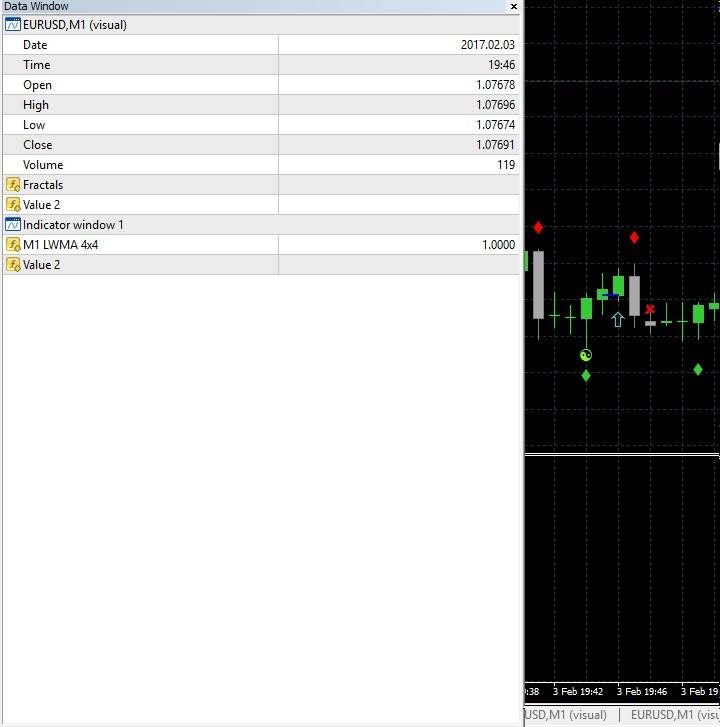The problem is that if there was an diamond the value LWMA 4x4 will show a value till there is a new/different diamond forming.

What could I do against that ? (I do not have the source code of the MA4x4 Indicator, I just got the indicator in .ex4 format.)
Is it possible to check if an diamond is drawn on that candle ?

Best regards,

salexes815

salexes:

So I figured out the problem, the problem is that if we have for example this setup:

The problem is that if there was an diamond the value LWMA 4x4 will show a value till there is a new/different diamond forming.

What could I do against that ? (I do not have the source code of the MA4x4 Indicator, I just got the indicator in .ex4 format.)
Is it possible to check if an diamond is drawn on that candle ?

Best regards,

salexes

Solved, thank you whroeder1 for your help!5

salexes:
Solved, thank you whroeder1 for your help!

Hello, Could you explain how you solve the problem?

Thanks5

hello sir

am in search for this indicators for long time